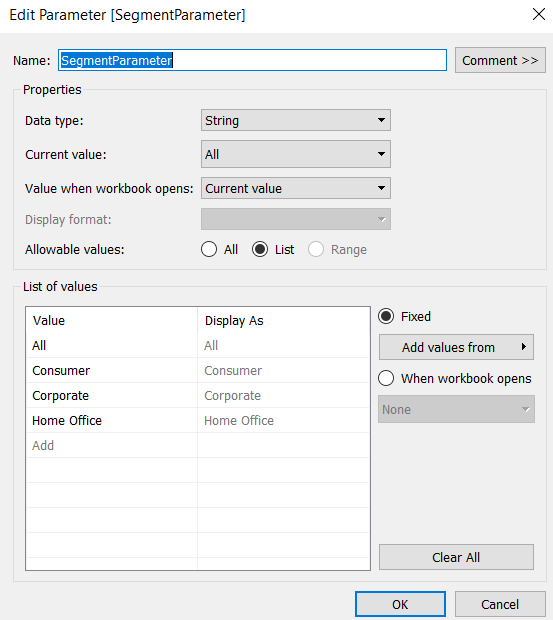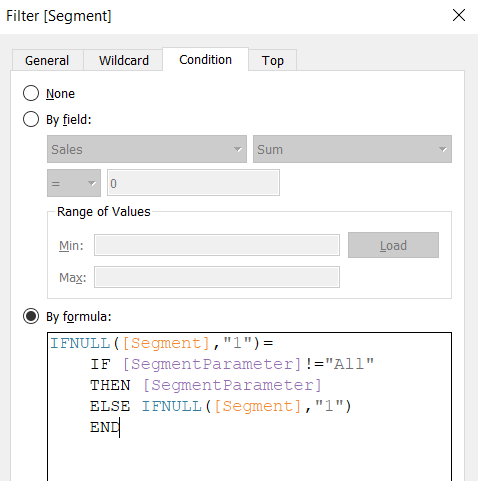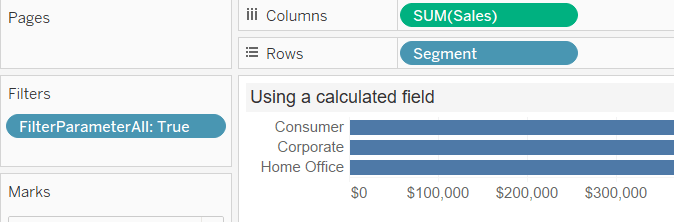The starting point for both of these “all to parameter” techniques is the same. Create a parameter that includes an All value. We will use this same parameter in both examples below.

The examples are using the Sample – Superstore data set that Tableau provides. We’re building a parameter filter on the Segment field.## All to a Tableau parameter using a Filter formula

Our parameter is filtering the Segment field. Therefore put the [Segment] field on the filters shelf.

Edit the [Segment] filter; select Condition and By formula.

Enter the following formula:

`IFNULL([Segment],"1")=IF [SegmentParameter]!="All" THEN [SegmentParameter] ELSE IFNULL([Segment],"1") END`That’s it, you have included an All in your Tableau parameter.

## All in a parameter using a calculated field

Another option is to use a calculated field instead of a filter formula.

First, create the calculated field.

`[FilterParameterAll]: [SegmentParameter] = 'All' OR [SegmentParameter] = [Segment]`

Next add the calculated field to the Filters shelf and set to True.Now the All is now fully operational in the parameter!

Both of these examples are on Tableau Public for you to download. Plus it is embedded below.